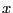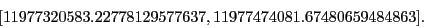Next: Sign of a polynomial Up: Evaluation of a polynomial Previous: Evaluation in centered form   Contents

Safe evaluation of a polynomial

Due to the numerical error in the coefficients and inthe evaluation of a polynomial atmay be incorrect. The following procedures enable to compute an interval which is guaranteed to include the true value:

INTERVAL Evaluate_Polynomial_Safe_Interval(int Degree,VECTOR &Coeff,REAL P);
INTERVAL Evaluate_Polynomial_Safe_Interval(int Degree,VECTOR &Coeff,INTERVAL P);
INTERVAL Evaluate_Polynomial_Safe_Interval(int Degree,INTERVAL_VECTOR &Coeff,REAL P);
INTERVAL Evaluate_Polynomial_Safe_Interval(int Degree,INTERVAL_VECTOR &Coeff,INTERVAL P);

For example for the Wilkinson polynomial at order 15 the evaluation for 15.1 leads to the interval:If "safe" intervals have been pre-computed (for example by using the procedure described in section 5.9.10) for the coefficients you may use:

INTERVAL Evaluate_Polynomial_Fast_Safe_Interval(int Degree,INTERVAL_VECTOR &Coeff,REAL P);
INTERVAL Evaluate_Polynomial_Fast_Safe_Interval(int Degree,INTERVAL_VECTOR &Coeff,INTERVAL P);
which are faster. If you want to use a centered form you may use:

INTERVAL Evaluate_Polynomial_Centered_Safe_Interval(int Degree,VECTOR &Coeff,REAL P);
INTERVAL Evaluate_Polynomial_Centered_Safe_Interval(int Degree,VECTOR &Coeff,INTERVAL P);
INTERVAL Evaluate_Polynomial_Centered_Fast_Safe_Interval(int Degree,
INTERVAL_VECTOR &Coeff,REAL P);
INTERVAL Evaluate_Polynomial_Centered_Fast_Safe_Interval(int Degree,
INTERVAL_VECTOR &Coeff,INTERVAL P);
in which the two last forms assume that you have pre-computed a safe value of the coefficients of the polynomial.

The evaluation of a polynomial with real coefficients at a complex point may be performed with:

INTERVAL_VECTOR Evaluate_Complex_Poly(int deg,
INTERVAL_VECTOR &Coeff, INTERVAL &XR,INTERVAL &XI)
where XR is the real part of the point and XI its imaginary part. This procedure returns an interval vector whose first element is the real part of the evaluation and the second element its imaginary part.Next: Sign of a polynomial Up: Evaluation of a polynomial Previous: Evaluation in centered form   Contents
Jean-Pierre Merlet 2012-12-20